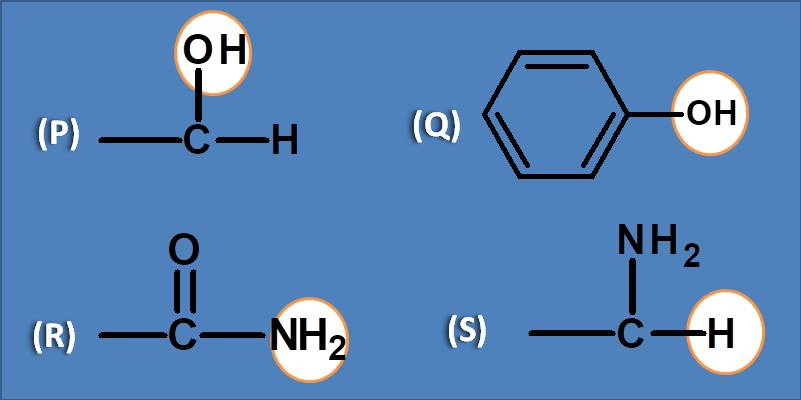1. Home
2. â€º
3. Test papers
4. â€º

# MCQ on NMR spectroscopy: Page-6

Given below is the splitting pattern of two neighbouring nuclei A and B in 1H NMR spectroscopy. Select the correct statement regarding their coupling constants.(A) J AB > J BA

(B) J BA > J AB

(C) J AB=J BA

(D) J AB=2 * J BA

Coupling constant will be more in

(A) Cis

(B) trans

(C) Geminal

(D) It depends on bond angle

Which of the following statement is TRUE

(A) Vicinal coupling depends on the bond angle

(B) Geminal coupling constants are independent of bond angle

(C) Vicinal coupling constants are always greater than germinal coupling constants

(C) Geminal coupling in alkenes is less than cis coupling

Consider the encircled protons in the following functional groups. Which of the proton among them will show more chemical shift?(A) P and Q

(B) R and S

(C) R only

(D) Q only

Which of the following corresponds to the notation A3B2X(A) Ethyl chloride

(B) Acetyl fluoride

(C) Ethyl fluoride

(D) Acetyl bromide